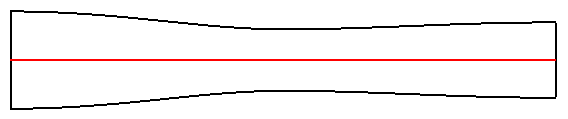NPARC Alliance CFD Verification and Validation Archive
V&V Home   >   Archive   >   Converging-Diverging Verification (CDV) Nozzle

## Converging-Diverging Verification (CDV) NozzleFigure 1. Converging-Diverging Verification (CDV) Nozzle (red is centerline).

### Introduction

The Converging-Diverging Verification (CDV) Nozzle is a verfication case involving the flow of inviscid, non-heat-conducting air through a converging-diverging nozzle. This is a classic one-dimensional, steady, compressible flow problem discussed in most compressible flow textbooks, such as Reference 1 by Anderson. This case allows the verification of a CFD code in the following manner:

• verify through comparison with analytic solutions
• verify mass conservation through a duct
• verify of constancy of total pressure through a duct (isentropic flow)
• verify consistency of axisymetric and three-dimensional flow domains

### Flow Description

This case involves steady, inviscid, non-heat-conducting flow through a converging-diverging nozzle. The plenum total pressure and total temperature are assumed constant. The values used in this case are presented in Table 1. The nature of the flow is determined by the exit static pressure. Three values of exit static pressure are examined which result in three types of flows:

1. subsonic, isentropic flow (pexit/pt=0.89)
2. supersonic flow with a normal shock in the diffusing section (pexit/pt=0.75)
3. supersonic, isentropic flow (pexit/pt=0.16)

For these exit static pressures, the flow is accelerated out the nozzle.

Plenum Total Pressure (psi) 1.0 100.0 0.89 0.75 0.16

### Geometry

The geometry is an axisymmetric converging-diverging duct. Figure 1 shows the general shape of the nozzle. It has an area of 2.5 in2 at the inflow (x = 0 in), an area of 1.0 in2 at the throat (x = 5 in), and an area of 1.5 in2 at the exit (x = 10 in). The nozzle area variation uses a cosine function and has the form

```      if ( x .lt. 5.0 ) then
area = 1.75 - 0.75 * cos( ( 0.2 * x - 1.0 ) * pi )
else
area = 1.25 - 0.25 * cos( ( 0.2 * x - 1.0 ) * pi )
endif
```

This nozzle geometry is from Reference 2, which also discusses some CFD computations of this nozzle.

### Comparison Data

The comparison data consists of static pressures and Mach number distributions along the centerline of the nozzle as computed from one-dimensional, steady, inviscid, compressible flow theory.

Table 2. Experimental static pressures in streamwise direction.
p/pt Static Pressure Mach Number
0.89 axial.Pex.p89.gen axial.Mex.p89.gen
0.75 axial.Pex.p75.gen axial.Mex.p75.gen
0.16 axial.Pex.p16.gen axial.Mex.p16.gen

### Computational Studies

Table 3. Computational studies peformed for the converging-diverging verification nozzle case.